=>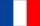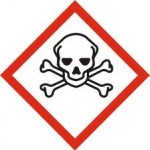Beware, this document contains equations

Having written a lot of posts in this blog, twitter or linkedIn, or due to the distribution of products and softwares on Theia or Github, I get about 10 questions per week in one of these channels, which I try to answer, even if not always at once.

Many questions are about reflectance values, such as:

• « when dividing the images by 10,000 the maximum value I saw was around 1.6, I guess the values are supposed to be within 0 and 1? »
• « I am trying to figure out if the TOA Reflectance range should be limited to 0 and 1 or not. I have read in two different posts that the TOA Reflectance should not be bigger than 1.The part that confuses me is that for many images I am using, the maximum pixel value is above 1 »
• Or, in a less diplomatic way from a VENµS user : « It means the CNES radiometric calibration process for Venus imagery has many bugs, starting from L1  all the way to L2 products with max surface reflectance values > 2.0 »

But the fact that reflectances should be lower than one is a common belief which is… false !

### Photometry

Let’s go back to physics :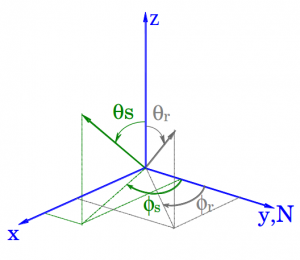Angle conventions, N is the North direction, in the text, we used the v subscript for viewing angles instead of r subscript).

a cold and passive surface does not emit light in the solar spectrum (or only negligibly), and only reflects some part of the incoming light. The ratio between the incoming light flux and the outgoing flux in all directions is known as the albedo, and it cannot be greater than one (see this post for a definition of fluxes, irradiance, radiance, and reflectance). But reflectance and albedo are not the same thing.

The Bidirectional reflectance is defined as :

$$\rho(\theta_{s},\phi_{s},\theta_{v},\phi_{v}) =\frac{\pi.L(\theta_{s},\phi_{s},\theta_{v},\phi_{v})}{Es.cos(\theta_{s})}$$

where L is the radiance, Es is the sun irradiance and $$\theta_s$$ is the solar zenith angle, $$\phi_s$$ is the sun azimuth angle, $$\theta_v$$ is the viewing zenith angle, and $$\phi_v$$ is the viewing azimuth angle.

The black sky albedo BSA, (or directional Hemispherical reflectance (DHR) is the ratio of incoming and outgoing light for a point source, such as the sun, with no atmosphere. The BSA is the integral of the reflectance in all directions (weighted by the cosine of the viewing angle, because we only account for the surface which is perpendicular to the observation direction, and by the sinus of viewing angle, as we integrate along crowns on the hemisphere).

$$BSA(\theta_{s},\phi_{s})=\int_{0}^{\pi/2} \int_{0}^{2. \pi} rho(\theta_{s},\phi_{s},\theta_{v},\phi_{v}) cos(\theta_v).sin(\theta_v)d\theta_v d\phi_v$$

Of course the total irradiance reflected by a surface in all directions is lower than the incoming sun irradiance, which means that the albedo is lower than one. Suppose you have a perfect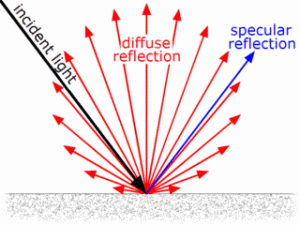lambertian surface, that scatters all the incoming light in a uniform way in all the upward directions (imagine a perfect white paper sheet). For such a surface, the reflectance is equal to one in all directions, and  the albedo, is equal to the reflectance.

Now imagine a perfect mirror, which also reflects all the incoming light, but only in the specular direction. In that case, the reflectance is not at all constant. It is null in all directions, except in a little cone that corresponds to the solid angle of the sun. The albedo is still one, but the reflectance in the specular cone is much greater than one :

$$\rho(\theta_{s},\phi_{s},\theta_{s},\phi_{s}+\pi) = \frac{2.\pi}{\omega_s}$$

Where $$\omega_s$$ is the sun solid  angle (and $$2.\pi$$) is the hemispherical solid angle.

As $$\omega_s=6.8 \times 10^{-5}$$, we get a reflectance of about $$10^{5}$$ for a perfect mirror, which is much greater than one. In practice, we have atmospheric effects, imperfect mirrors, and detectors that saturate, so we do not observe such high reflectances wth our satellites, but we can still find reflectances greater than 1.

### Usual cases of observations of reflectances greater than one :

#### Specular reflections

For instance, a window roof that reflects the sun right right to the satellite camera, can have a reflectance much higher than one. It is the case for the images below, for which a user wrongly complained that our products were providing maximum surface reflectance values greater than 2, and therefore were completely bugged (which is not the case, or not completely the case 😉 )

#### Slope effect on snow mountains

Another phenomenon contributes to observing reflectances larger than one :

A snow covered pixel in a mountain facing the sun can also reach values above one. This effect is due to the fact that reflectance is normalized by the cosine of sun zenith angle (angle between sun

direction and vertical). But in the case of a slope, it should be in fact normalized by the cosine of the incidence angle, which is the angle between sun direction and the normal direction to the slope. Sen2cor and MACCS have a “terrain correction” to correct for this effect.

####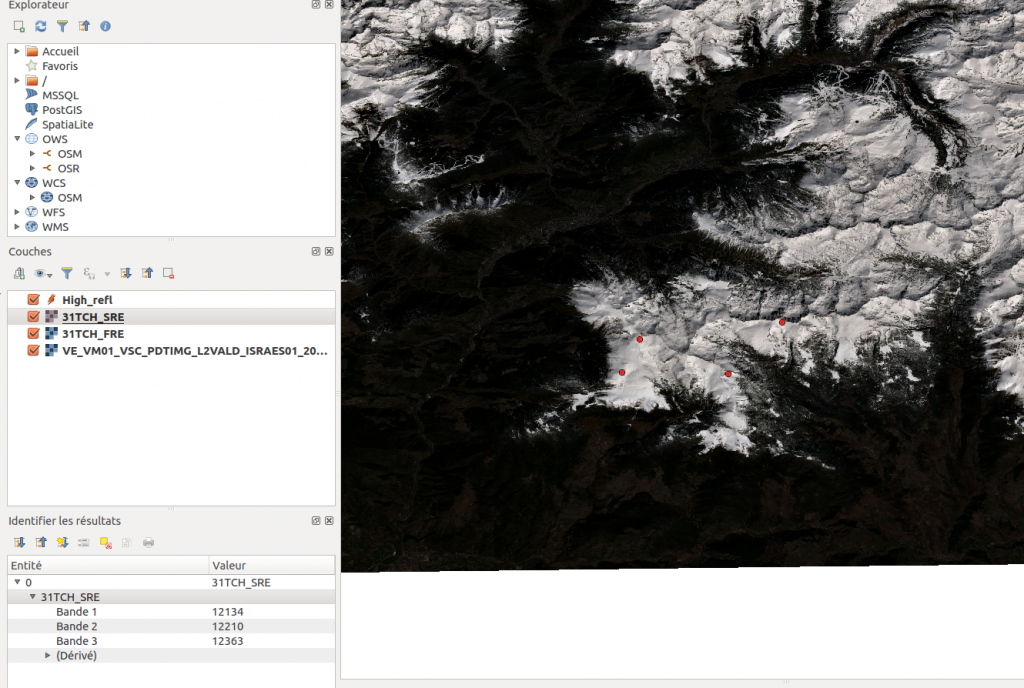Slope effect on clouds

Thick clouds can also have very high reflectances, and the same effect as above can be observed for slopes in the cloud surface that face the sun. But we are not able to correct it as it would mean having an elevation model of the cloud.

### References:

Nicodemus, F.E.; J.C. Richmond; J.J. Hsia (October 1977). « Geometrical Considerations and Nomenclature for Reflectance » (PDF). NATIONAL BUREAU OF STANDARDS. Retrieved 8 April 2013.

Schaepman-Strub, G., Schaepman, M. E., Painter, T. H., Dangel, S., & Martonchik, J. V. (2006). Reflectance quantities in optical remote sensing—Definitions and case studies. Remote sensing of environment, 103(1), 27-42.

## 2 thoughts on “[How it works] Can reflectances be greater than 1 ?”

1.Eyal Ben Dor dit :

perhaps this is a problem only of absolute reflecance relative calibration and not a specular reflection. The specular explanation need to be followed by more solid measurements and evidences

1.Olivier Hagolle dit :

Hi Eyal,
if you want to try to prove that a specular reflection on a rooftop cannot be greater than one, you’re welcome.
Olivier

Ce site utilise Akismet pour réduire les indésirables. En savoir plus sur comment les données de vos commentaires sont utilisées.